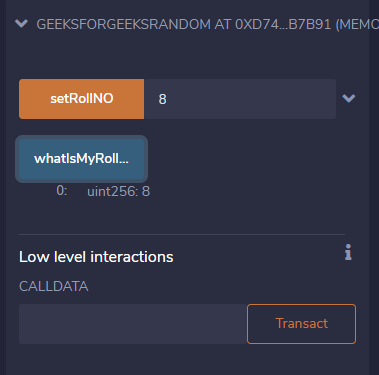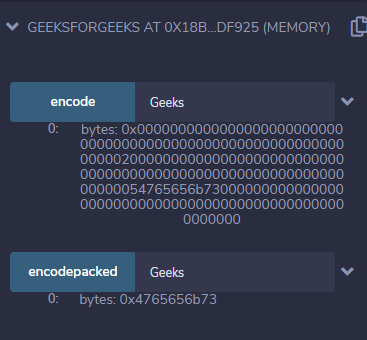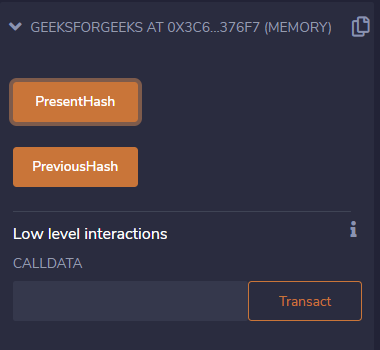Related Articles
Solidity – Special Variables
• Last Updated : 18 Sep, 2020

There exist special variables and functions in solidity which exist in the global namespace and are mainly used to provide information about the blockchain or utility functions. They are of two types:

1) Block and Transaction Properties:

Note:

• The values of all members of msg can change for every external function call.
• block.timestamp, now and blockhash as a source of randomness are not secure. Timestamp and the blockhash can be influenced by the miners.

2) ABI encoding and decoding functions:

Example #1: In the below example, a contract is created to demonstrate msg.sender as a secure way to store the roll number.

## Solidity

 `// Solidity program to  ` `// demonstrate msg.sender ` `pragma solidity ^0.6.6; ` ` `  `// Creating a smart contract ` `contract` `GeeksForGeeksRandom  ` `{ ` `    ``// Creating a mapping ` `    ``mapping (address => uint) rollNo; ` `     `  `    ``// Defining a function to use  ` `    ``// msg.sender to store roll no. ` `    ``function setRollNO(uint _myNumber) ``public`  `    ``{ ` `        ``// Update our 'rollNo' mapping  ` `        ``// to store '_myNumber' under  ` `        ``// 'msg.sender' ` `        ``rollNo[msg.sender] = _myNumber; ` `    ``} ` `     `  `    ``// Defining a function to ` `    ``// return the roll no. ` `    ``function whatIsMyRollNumber() ` `             ``public` `view returns (uint)  ` `    ``{ ` `        ``// Retrieve the value stored  ` `        ``// in the sender's address ` `        ``// Will be `0` if the sender  ` `        ``// hasn't called `setRollNO` yet ` `        ``return` `rollNo[msg.sender]; ` `    ``} ` `}`

Output:Example#2: In the below example, a contract is created with a function that demonstrates the variable abi.encode.

## Solidity

 `// Solidity program to  ` `// demonstrate abi.encoding ` `pragma solidity ^0.6.6; ` ` `  `// Creating a contract ` `contract` `GeeksForGeeks  ` `{     ` `    ``// Defining a function  ` `    ``// to use abi.encode() ` `    ``//It does padding to bytes ` `    ``function encode(string memory g)  ` `             ``public` `pure returns(bytes memory) ` `    ``{ ` `        ``return` `abi.encode(g); ` `    ``} ` `     `  `    ``// encodepacked returns values in  ` `    ``// a packed way without padding ` `    ``function encodepacked(string memory g) ` `             ``public` `pure returns(bytes memory) ` `    ``{ ` `         ``return` `abi.encodePacked(g); ` `    ``} ` `}`

Input:

```Geeks
```

Output:Example#3: In the below example, a contract is created to demonstrate the special variables block.number and blockhash.

## Solidity

 `// Solidity program to  ` `// demonstrate block.number ` `// and blockhash ` `pragma solidity ^0.4.0; ` ` `  `// Creating a contract ` `contract` `GeeksForGeeks  ` `{    ` `    ``// Declaring state variables ` `     `  `    ``// BlockNumber ` `    ``uint  BNumber;  ` `     `  `    ``// Hash of current block ` `    ``bytes32  BHashPresent;  ` `     `  `    ``// Hash of Previous Block ` `    ``bytes32  BHashPrevious;  ` ` `  `    ``// Defining a function to  ` `    ``// return hasdh value of  ` `    ``// the current block ` `    ``function PresentHash() ` `             ``public` `returns(bytes32) ` `    ``{ ` `        ``BNumber = block.number; ` `        ``return` `BHashPresent =  ` `               ``block.blockhash(BNumber); ` `    ``} ` `     `  `    ``// Defining a function to  ` `    ``// return the hash value of ` `    ``// the previous block ` `    ``function PreviousHash()  ` `             ``public` `returns(bytes32) ` `    ``{ ` `        ``BNumber = block.number; ` `        ``return` `BHashPrevious =  ` `               ``block.blockhash(BNumber - 1); ` `    ``}   ` `}`

Output:My Personal Notes arrow_drop_up
Recommended Articles
Page :